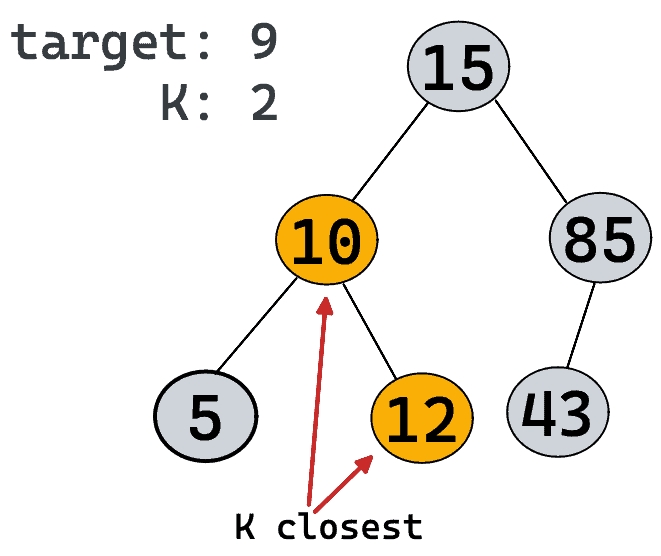# Closest Binary Search Tree Value IILeetcode 272. Closest Binary Search Tree Value II

##### Problem

Given the `root` of a binary search tree, a `target` value, and an integer `k`, return the `k` values in the BST that are closest to the `target`. You may return the answer in any order.

You are guaranteed to have only one unique set of `k` values in the BST that are closest to the `target`.

Example 1:

```Input: root = [4,2,5,1,3], target = 3.714286, k = 2
Output: [4,3]
```

Example 2:

```Input: root = , target = 0.000000, k = 1
Output: 
```

Constraints:

• The number of nodes in the tree is `n`.
• `1 <= k <= n <= 104`.
• `0 <= Node.val <= 109`
• `-109 <= target <= 109`

Follow up: Assume that the BST is balanced. Could you solve it in less than `O(n)` runtime (where `n = total nodes`)?

##### Solution

The straight forward solution of this problem is to use a heap (a.k.a priority queue).

We can run an inorder traversal of the tree. That way, we will get the values in sorted order. During that process, we can save the absolute difference between target and node value to the heap. Also, we need to have the node value. That’s why it is good to have a pair of absolute difference and node value [something like {difference, val}] in the heap. Also, when we push those pairs in heap, we will check if heap size is greater than K. If it is, we will remove the last element from heap.

Below is the code that implements this idea.

###### C++ Code
```/**
* Definition for a binary tree node.
* struct TreeNode {
*     int val;
*     TreeNode *left;
*     TreeNode *right;
*     TreeNode() : val(0), left(nullptr), right(nullptr) {}
*     TreeNode(int x) : val(x), left(nullptr), right(nullptr) {}
*     TreeNode(int x, TreeNode *left, TreeNode *right) : val(x), left(left), right(right) {}
* };
*/
class Solution {
public:
void inorder(TreeNode* root, double target, int k, priority_queue<pair<double, int>>& pq){
if(root == NULL)
return;
inorder(root->left, target, k, pq);
pq.push({abs(target - root->val), root->val});
if(pq.size() > k)
pq.pop();
inorder(root->right, target, k, pq);
}

vector<int> closestKValues(TreeNode* root, double target, int k) {
vector<int> ans;
if(root == NULL)
return ans;
priority_queue<pair<double, int>> pq;
inorder(root, target, k, pq);
while(!pq.empty()){
ans.push_back(pq.top().second);
pq.pop();
}
return ans;
}
};```
##### Conclusion

Hope you understood this solution. If not, please comment below which part was not so clear.

Happy Coding! 🙂